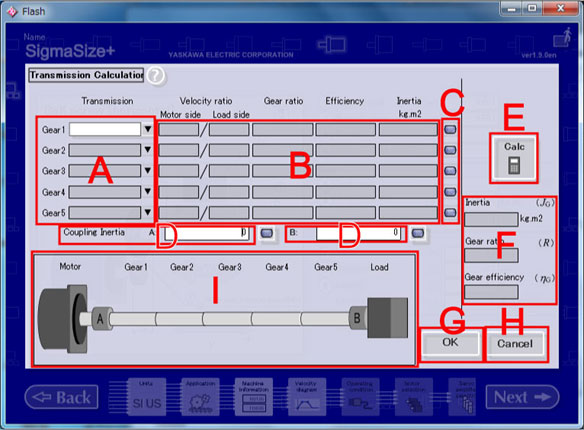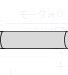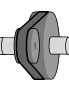Wizard sizing / Transmission calculation

Transmission calculation

Calculate "a moment of inertia", "a gear ratio" and a "gear efficiency" of transmission that constructed with "a gear", "a pulley", and "a gearbox".
When data was load with Data load/ delete or Machine information, or Transmission already calculated, parameters that were already finished setting are displayed.

[Operation Procedure]

1. Select a transmission.
2. Input parameter according to the selected transmission.
3. Input coupling moment of inertia that connect motor with transmission and connect transmission and load.
4. Click "Calc".
The value of Inertia, Gear ratio and Gear efficiency is calculated and displayed at that point.
5. "OK" is clicked
it returns to the Machine information and displays the calculation result.
When "Cancel" is clicked, it returns to the Machine information after deleting input data.
Note 1)Handling of coupling moment of inertia that connects between transmission.
Add to the load side coupling moment of inertia of that connects between transmission.

Note 2)Handling of "Gear" between transmission.
Moment of inertia of "Gear" is value in which moment of inertia on load side where gear ratio was considered was added to motor side.
Example)In the case ,a gear of the load side engages with a gear of the motor side directly
 Momemt of inertia at Gear1 JG1 Moment of inertia at the motor side JGm1 Moment of inertia at the load side JGl1 Gaer ratio RG1 JG1  =  JGm1  + JGl1 / RG12
The present, it is impossible for calculating the moment of inertia of "gear" mechanism in SigmaSize+ directly
You can demand it with this "Transmission calculation" function as follows.
1. Invalidate all the Gear2 from Gear5.
2. Input rotate speed ration of gear and efficiency each at Gear1.
3. Input 0 at column of Gear1's moment of inertia.
4. Input the motor side gear moment of inertia into coupling moment of inertia A.
5. Input the load side gear moment of inertia into coupling moment of ineartia B.
6. Click "Calc" button
The displayed mement of inertia (JG)becomes the "Gear" moment of inertia.

[Screen Structure]Transmission calculation screen

1. Transmission
Select transmission from combo box.

2. Data input column
Input item according to the selected transmission.

 Gear Velocity ratio, Effeciency and Inertia Pulley Velocity ratio, Effeciency and Inertia GearBox Gear ratio, Effeciency and Inertia
* Input moment of inertia seen from the input axis of the transmission.

3. Inertia calculation button
Inertia calculation is open.

4. Coupling moment of inertia column
Input the coupling moment of inertia that connects motor, transmission and load.

5. Data output column
Inertia, gear ratio and gear efficiency.

6. Calc button
Calculates inertia, gear ratio and gear efficiency.

7. OK button
Returns to the Machine information and displays the calculation result.

8. Cancel button
Returns to the Machine information detailed Machine Information, and then deletes the selected rigid body and input data.

9. Transmission chart
Selected transmission is shown in the chart.

Non selection Gear Pulley Gear Box